0

# 利用yolov5进行目标检测，并将检测到的目标裁剪出来

## 利用yolov5进行目标检测，并将检测到的目标裁剪出来

``````https://www.bilibili.com/video/BV1tf4y1t7ru/spm_id_from=333.999.0.0&vd_source=043dc71f3eaf6a0ccb6dada9dbd8be37
``````

``````python --classes 0
``````

``````python --classes0 --save-txt
``````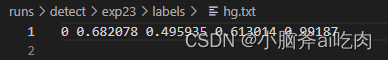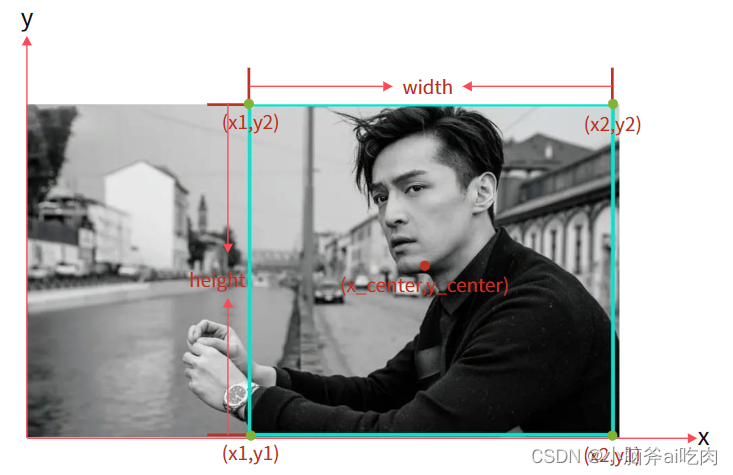``````x1=x_center-width/2
x2=x_center+width/2
y1=y_center-height/2
y2=y_center+height/2
``````

``````x1=（x_center-width/2）*整张图片的宽度(注：是整张图片的宽度不是目标的宽度,这里的宽度就是像素宽度)x2=(x_center+width/2)*整张图片的宽度
y1=(y_center-height/2)*整张图片的高度（注：是整张图片的高度不是目标的高度,这里的高度就是像素高度）
y2=(y_center+height/2)*整张图片的高度
``````

``````import os
import cv2

defmain():#图片路径
img_path ='./data/images/hg.jpg'#txt文件路径
label_path ='./runs/detect/exp23/labels/hg.txt'# 读取图片，结果为三维数组
w = img.shape# 图片高度(像素)
h = img.shape# 打开文件，编码格式'utf-8','r+'读写
f =open(label_path,'r+', encoding='utf-8')# 读取txt文件中的第一行,数据类型str
msg = line.split(" ")
x1 =int((float(msg)-float(msg)/2)* w)# x_center - width/2
y1 =int((float(msg)-float(msg)/2)* h)# y_center - height/2
x2 =int((float(msg)+float(msg)/2)* w)# x_center + width/2
y2 =int((float(msg)+float(msg)/2)* h)# y_center + height/2print(x1,",", y1,",", x2,",", y2)#裁剪
img_roi = img[y1:y2,x1:x2]
save_path='./cutpictures/hg.jpg'
cv2.imwrite(save_path,img_roi)if __name__ =='__main__':
main()
``````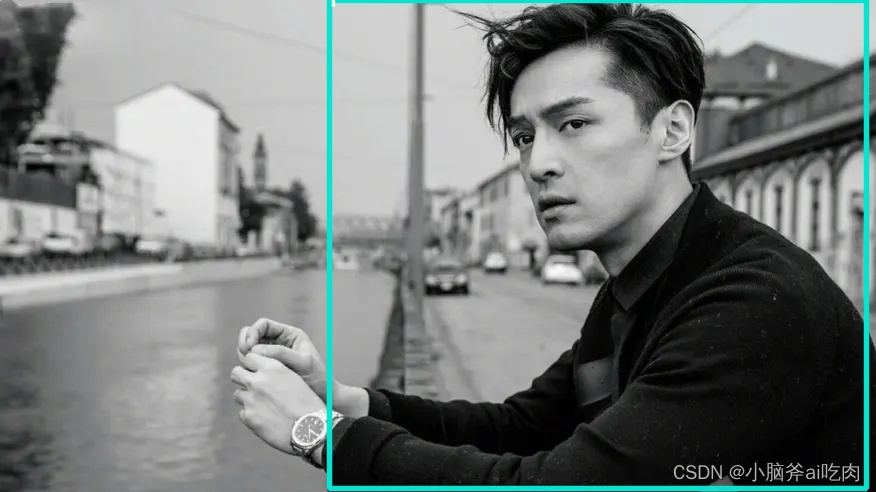``````f.readlin()
``````

``````f.readlines()
``````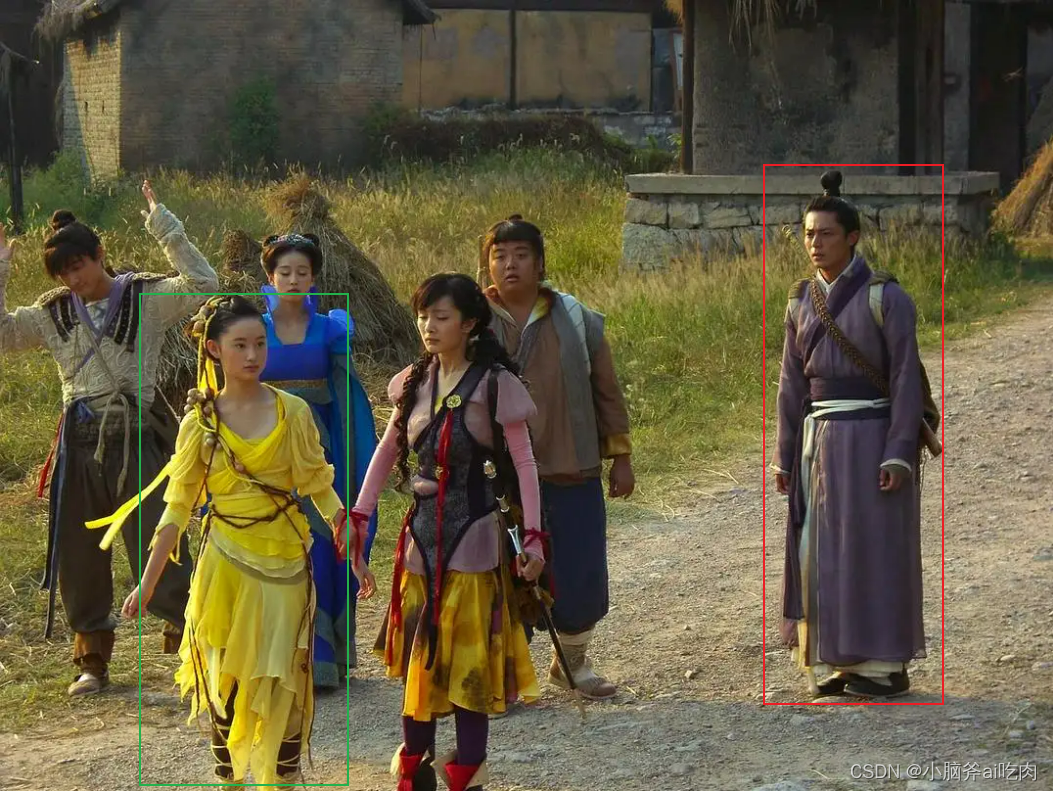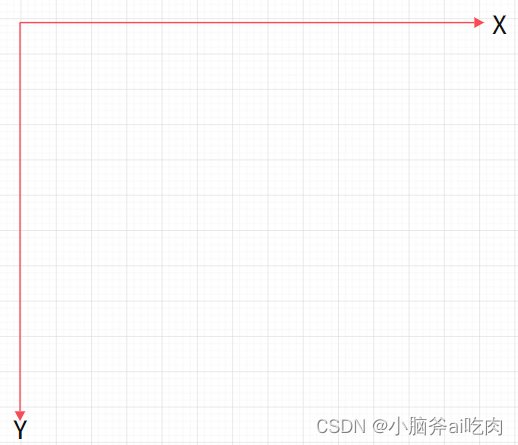### “利用yolov5进行目标检测，并将检测到的目标裁剪出来”的评论:

##### 关于作者##### overfit同步小助手